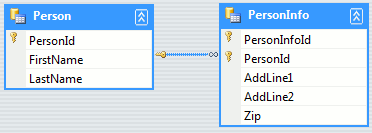## Using Extension Methods With Typed Datasets

DataTable is one of the most widely used classes in .NET framework. In data layers of a typical ADO.NET application one can see lot of code to work with DataTable and DataRow. In this article I discuss how to do some common tasks involving datasets in more fashionable manner, in LINQ style. This is not a tutorial on LINQ or extension methods or typed Datasets.

I am assuming that most of you already know lot more about them than I do (if not, don't worry there are always late starters like me :-) ). So, the first question is why only 'typed' datasets ? why isn't this article covering normal datasets. To answer this question, let use create a typed dataset using VS2008 I have created the following dataset, it has two tables:In .NET 3.5 all tables of a typed dataset inherit TypedTableBase<T>, which in turn implements IEnumerable<T>. If you have been reading about .NET 3.5 stuff, you will realize that this is the interface that has many powerful extension methods defined for it. This enables us to use LINQ's extension methods with our DataTable classes. If this doesn't excite you, go thorough the code snippets below:

### 1. Filtering Rows

The traditional way:
```DataRow  pr = p.Person.Select("Firstname ='a'");
```
With extension methods:
```var prst = p.Person.Where(n => n.FirstName=="a").ToArray<Persons.PersonRow>();
```

### 2. Joining tables to get data

Suppose we want to get all PersonInfo rows for persons whose first name contains 'a'. Now imagine writing C# code for that without use extension methods, and compare it with this:
```var onlyinfo = p.Person.Where(n=>n.FirstName.Contains('a')).Join(p.PersonInfo,
n => n.PersonId,
m => m.PersonId,
(n, m) => m).ToArray<Persons.PersonInfoRow>();
```

### 3. Summing up a particular column

To get sum of all Ids (kinda of vague but just for example :-))
```int sumofids = p.Person.Sum(n => n.PersonId);
```

### 4. Getting a set of continus rows

Get row no. 3, 4 and 5
```var x= p.Person.Skip(2).Take(3);
```

### 5. Sorting by a particular field

Sorting by last name
```var o = p.Person.OrderBy(n => n.LastName);
```
Sort by "LastName,FirstName"
```var y = p.Person.OrderBy(n => n.LastName+","+n.FirstName).Reverse();

```

### 6. Join using LINQ syntax

Here we join two tables using LINQ syntax. What the query does is fetches custom resultset for all persons whose PersonId < 5
```var tm = from l in p.Person
join q in p.PersonInfo
on l.PersonId equals q.PersonId
where l.PersonId < 5
select new { l.PersonId, q.PersonInfoId, l.FirstName, l.LastName };

var myarr = tm.ToArray();

Console.WriteLine("{0} {1} {2} {3}",
myarr.FirstName, myarr.LastName,
myarr.PersonId,myarr.PersonInfoId);

```
These were just some examples of what you can do with typed datasets .NET 3.5. In many cases, using extension methods will reduce the amount of code you write drastically.

Copyright (c) 2007-2019 Ashish Patil . Please read FAQ for more details.

comments powered by Disqus